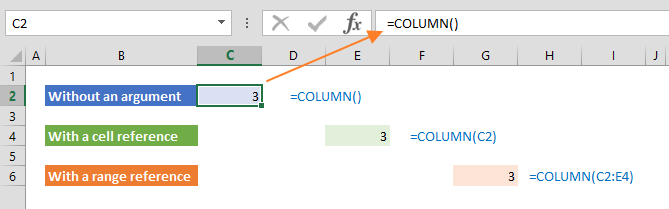The Excel COLUMN function is a Lookup & Reference formula that returns the column number of a reference. In this guide, we’re going to show you how to use the Excel COLUMN function and also go over some tips and error handling methods.

# Supported versions

• All Excel versions

# Syntax

COLUMN([reference])

# Arguments

 [reference] Optional. A valid reference.

Examples

The COLUMN function returns the column number where the formula is entered, if no arguments is entered.

=COLUMN()

If a valid cell reference is entered as an argument, the return value will be the number of the reference column.

=COLUMN(C2)

If you enter a range reference, you will get the number of the top column. When dynamic arrays are introduced, you will get the numbers of all columns in the range reference.

=COLUMN(C2:E4)## Generating number series

A common way of using the Excel COLUMN function is finding the column number of a reference. Using the function in multiple adjacent columns, you can get a series of numbers. In our example, we placed the COLUMN function in cell in column 3 (column C) and subtracted 2 from the column number. This gives us 1 for the column 3. If we copy same formula to the column I, we get a series of numbers from 1 to 7.

=COLUMN()-2# Tips

• Use the COLUMNS function if you need to count the number of columns in a reference.
• To get the row number of a reference, use the ROW function.